# How to use the DAYS function in Excel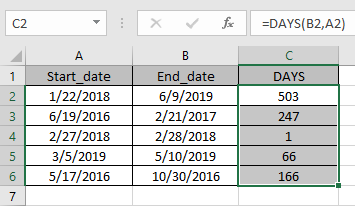In this article, we will learn How to use the DAYS function in Excel.

Number of days between two dates

Date values in Excel are stored as numbers. To take the difference between two date values just use the subtract operator (-) between the two given values. For example getting the number of days between the patient admitted to a patient detarted in a hospital. use the DAYS function to do the same task using cell reference. Let's learn more about DAYS function syntax and an example to illustrate the function usage.

DAYS Function in Excel

DAYS function gets a number which is the count of days between two given dates.

Syntax:

 =DAYS (end_date, start_date)

Start_date : from the date

End_date : till the date

Example :

All of these might be confusing to understand. Let's understand how to use the function using an example. Here we have some date values and we need to find the number of days between them.Use the formula.

 =DAYS(B2,A2)

B2 : end_date

A2 : start_dateYou can see the formula returns a number, the total days elapsed between the two dates.

Now copy the formula to other cells using shortcut key Ctrl + D.Use the above function formulas to get the days between dates in Excel.

Alternate method

Excel stores dates as numbers, a simple mathematical formulation explained. So just need to subtract the two dates it will get the days between the two dates.

 =later_date - earlier date

Or

 =IF(date1>date2, date1 - date2, date2 - date1)

Use the below formula, if you don’t know which date to put first.

Let’s understand this function using it an example.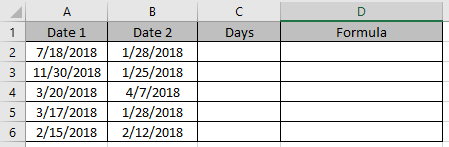Use the formula in C2 and D2 cells.

 =A2-B2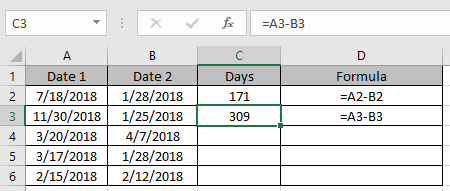This formula gets you negative results if the date1 < date2.

If you don’t want to care which is smaller, just use the below formula in remaining cells

 =IF(A4>B4, A4-B4, B4-A4)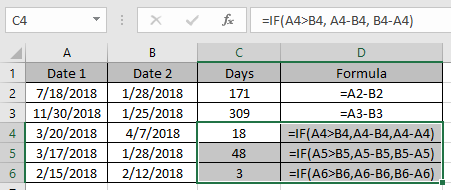Use the above function formulas to get the days between dates in Excel

DATEDIF function to get the number of days between two dates

Example :

All of these might be confusing to understand. Let's understand how to use the function using an example.

Here we have some examples to test the formula. Here given the start date and end date, we need to calculate the difference in days between dates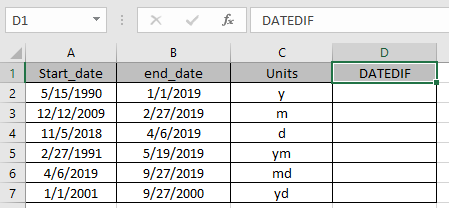Use the Formula:

 =DATEDIF (A2, B2, C2)

A2 : first date argument given as cell reference

B2 : Second date argument given as cell reference

C2 : specified unit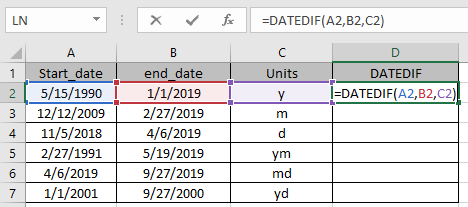Marked boxes while using the function shows us that argument to the function given as cell reference.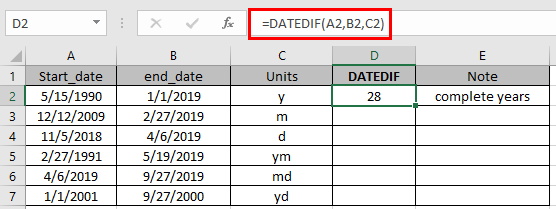The function returns the complete calendar years between the dates using the “y”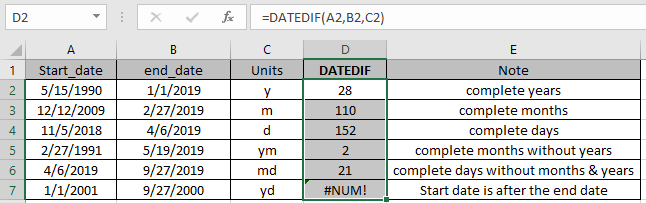As you can see the function returns the values.

You can also use the NETWORKDAYS function to get only the working number of days between the two given dates

Here are all the observational notes using the DAYS function in Excel
Notes :

1. Excel stores dates as serial numbers and is used in calculation by the function. So it’s recommended to use dates as cell reference or using the DATE function instead of giving direct argument to the function. Check the validation of date values if the function returns #VALUE! Error.
2. DAYS function can also subtract the two given numbers. Careful using the function
3. The date in excel must be in valid format
4. Unit argument in DATEDIF function can be given as cell reference or directly to the function in double quotes.
5. Start_date must be before the end_date or else the function will give #NUM! error
6. The function gives #VALUE! Error if the date is not a valid date.

Hope this article about How to use the DAYS function in Excel is explanatory. Find more articles on calculating values and related Excel formulas here. If you liked our blogs, share it with your friends on Facebook. And also you can follow us on Twitter and Facebook. We would love to hear from you, do let us know how we can improve, complement or innovate our work and make it better for you. Write to us at info@exceltip.com.

Related Articles :

How to use the NETWORKDAYS function in Excel : Returns the working days between the two given dates using the NETWORKDAYS function.

How to use the WORKDAYS function in Excel : Returns a workday date value after days added to the start_date using the WORKDAYS function in Excel.

Count holidays between dates in Excel : count non working days between the two given dates using the DATEDIF and NETWORKDAYS function in Excel.

Extract days between dates ignoring years in Excel : count the days between the two dates using different functions and mathematical operation in Excel

Count Birth Dates By Month in Excel : count the number of dates lying in a particular month using the SUMPRODUCT and MONTH function in Excel.

SUM price by weekdays in Excel : sum price values corresponding to the number of dates in a particular weekday using the SUMPRODUCT and WEEKDAY function in Excel.

Popular Articles :

How to use the IF Function in Excel : The IF statement in Excel checks the condition and returns a specific value if the condition is TRUE or returns another specific value if FALSE.

How to use the VLOOKUP Function in Excel : This is one of the most used and popular functions of excel that is used to lookup value from different ranges and sheets.

How to use the SUMIF Function in Excel : This is another dashboard essential function. This helps you sum up values on specific conditions.

How to use the COUNTIF Function in Excel : Count values with conditions using this amazing function. You don't need to filter your data to count specific values. Countif function is essential to prepare your dashboard.

1."hi
dear sir,
i just want to know that is it any formula which can we delete duplicate record in selected range of cell's and restrict a cell does not take duplicate records.
Thanks"

2.How can i re-name rows (1,2,3,..) and columns (a,b,c,..) to a custom setting

Terms and Conditions of use

The applications/code on this site are distributed as is and without warranties or liability. In no event shall the owner of the copyrights, or the authors of the applications/code be liable for any loss of profit, any problems or any damage resulting from the use or evaluation of the applications/code.